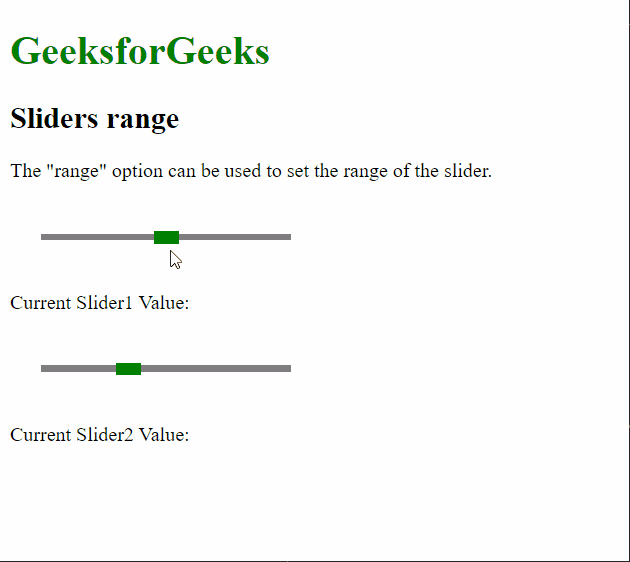Related Articles
script.aculo.us Sliders range Option
• Last Updated : 27 Nov, 2020

The script.aculo.us library is a cross-browser library that aims at improving the user interface of a website. The Slider controls are thin tracks that allow the user to input values. It is done by defining a range of values that can be selected by the user by dragging the handle to the appropriate value.

The Sliders range is used to define the range of the slider. The slider handle can only move within the value defined for both ends. The range has to be specified using \$R(min, max) syntax, where min denotes the minimum value of the slider and max denotes the maximum value.

Syntax:

`{ range: \$R( min, max ) }`

Values: This option has two values as mentioned above and described below:

• min: This is a number that denotes the minimum value of the range of the slider.
• max: This is a number that denotes the maximum value of the range of the slider.

Example:

 ```<``html``>``<``head``>``  ````  ``<``script` `type``=``"text/javascript"` `          ``src``=``"prototype.js"``>``  ````  ``<``script` `type``=``"text/javascript"``          ``src``=``"scriptaculous.js?load = slider"``>``  ```` ` `  ````  ``<``style` `type``=``"text/css"``>``    ``.track {``      ``width: 200px;``      ``background-color: gray;``      ``height: 5px;``      ``position: relative;``    ``}`` ` `    ``.track .handle {``      ``width: 20px;``      ``height: 10px;``      ``background-color: green;``      ``cursor: move;``      ``position: absolute;``      ``top: -2px;``    ``}`` ` `    ``.pad {``      ``padding: 25px;``    ``}``  ``````<``body``>``  ``<``p``>``  ``<``h1` `style``=``"color: green;"``>``    ``GeeksforGeeks``  ````  ``<``h2``>Sliders range``  ``<``p``>``    ``The "range" option can be used to``    ``set the range of the slider.``  ```` ` `  ``<``div` `class``=``"pad"``>``    ``<``div` `id``=``"track-hor"` `class``=``"track"``>``      ``<``div` `id``=``"handle-hor"` `class``=``"handle"``>``      ````    ````  ````  ``<``p``>Current Slider1 Value: ``    ``<``span` `id``=``"out1"``>``  ````  ``<``div` `class``=``"pad"``>``    ``<``div` `id``=``"track2-hor"` `class``=``"track"``>``      ``<``div` `id``=``"handle2-hor"` `class``=``"handle"``>``      ````    ````  ````  ``<``p``>Current Slider2 Value: ``    ``<``span` `id``=``"out2"``>``  ````  ``<``script` `type``=``"text/javascript"``>``    ``new Control.Slider('handle-hor',``      ``'track-hor', {`` ` `      ``// Setting the range of the slider``      ``// from 0 to 100``      ``range: \$R(0, 100),``       ` `      ``sliderValue: 50,`` ` `      ``onSlide: (val) => {``        ``document.querySelector("#out1")``                ``.textContent = val;``      ``}``    ``});`` ` `    ``new Control.Slider('handle2-hor',``      ``'track2-hor', {`` ` `      ``// Setting the range of the slider``      ``// from 20 to 50``      ``range: \$R(20, 50),``       ` `      ``sliderValue: 30,`` ` `      ``onSlide: (val) => {``        ``document.querySelector("#out2")``                ``.textContent = val;``      ``}``    ``});``  ```````

Output:My Personal Notes arrow_drop_up